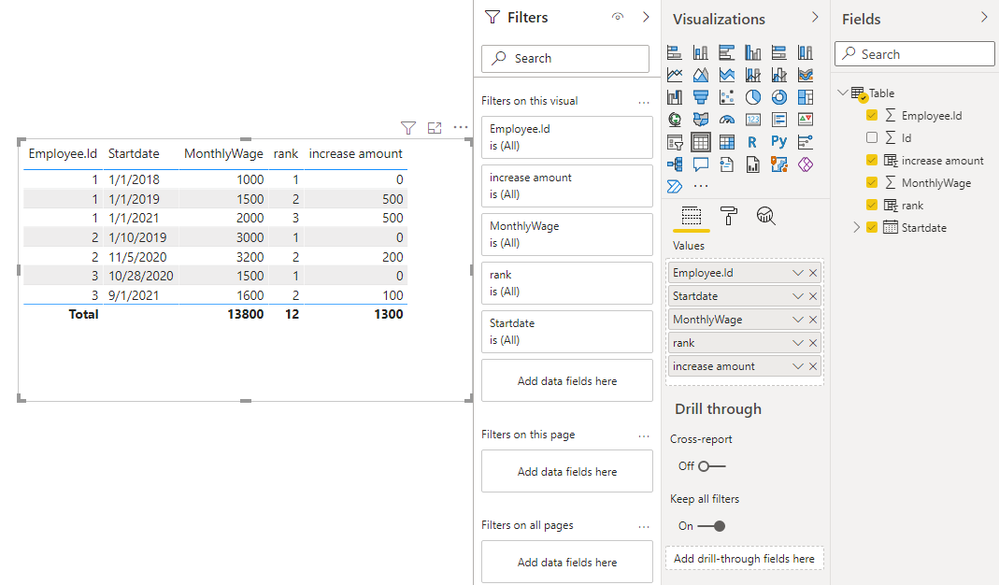cancel
Showing results for
Did you mean:

Fabric is Generally Available. Browse Fabric Presentations. Work towards your Fabric certification with the Cloud Skills Challenge.Anonymous
Not applicable

## find 2 latest wages by employee and calculate increase amount

Hi, I have a really tricky calculation for me that I can't resolve. I have a wage table where is all wages of employees. I need to calculate how much have the wage increased. There can be multiple wage increases/employee and it has to be calculated from the two latest agreement.

For example Employee.Id 1: Calculate(sum(monthlywage1-monthlywage2) where employee.id = 1 and monthlywage1=latestwage and monthlywage2 =secondlatest wage.

 Id Employee.Id Startdate MonthlyWage 1 1 01.01.2018 1000 2 2 10.01.2019 3000 3 1 01.01.2019 1500 4 2 05.11.2020 3200 5 1 01.01.2021 2000 6 3 28.10.2020 1500 7 3 01.09.2021 1600
1 ACCEPTED SOLUTIONCommunity Support

Hi @Anonymous ,

You can create two calculated columns

``````rank =
RANKX (
FILTER ( 'Table', [Employee.Id] = EARLIER ( 'Table'[Employee.Id] ) ),
[Startdate],
,
ASC,
DENSE
)
``````
``````increase amount =
VAR _amount =
CALCULATE (
SUM ( 'Table'[MonthlyWage] ),
FILTER (
'Table',
[Employee.Id] = EARLIER ( 'Table'[Employee.Id] )
&& [rank]
= EARLIER ( 'Table'[rank] ) - 1
)
)
RETURN
IF ( ISBLANK ( _amount ), 0, [MonthlyWage] - _amount )
``````Best Regards,

Stephen Tao

If this post helps, then please consider Accept it as the solution to help the other members find it more quickly.

4 REPLIES 4Community Support

Hi @Anonymous ,

You can create two calculated columns

``````rank =
RANKX (
FILTER ( 'Table', [Employee.Id] = EARLIER ( 'Table'[Employee.Id] ) ),
[Startdate],
,
ASC,
DENSE
)
``````
``````increase amount =
VAR _amount =
CALCULATE (
SUM ( 'Table'[MonthlyWage] ),
FILTER (
'Table',
[Employee.Id] = EARLIER ( 'Table'[Employee.Id] )
&& [rank]
= EARLIER ( 'Table'[rank] ) - 1
)
)
RETURN
IF ( ISBLANK ( _amount ), 0, [MonthlyWage] - _amount )
``````Best Regards,

Stephen Tao

If this post helps, then please consider Accept it as the solution to help the other members find it more quickly.Anonymous
Not applicable

Hi,

Great this works like a charm :)! Thank you very much!Super User

@Anonymous ,

Meausre =
var _max = calculate(Max(Table[Startdate]), allexcept(Table, Table[Employee.ID]))
var _max2 = calculate(MaxX(Filter(Table, Table[Startdate]<_max),Table[Startdate] ), allexcept(Table, Table[Employee.ID]))
return
calculate(sumX(values(Table[employee.id]) , calculate(sum(Table[MonthlyWage]), filter(Table, Table[Startdate]=_max)) -calculate(sum(Table[MonthlyWage]), filter(Table, Table[Startdate]=_max1))))Anonymous
Not applicable

Hi @amitchandak for some reason the calculation returns incorrect result. for example previous wage has been 1337 and new wage is 3000. The result should be 1663(the difference between current and previous wage) but calculation returns -1337Announcements#### Power BI Monthly Update - November 2023

Check out the November 2023 Power BI update to learn about new features.#### Fabric Community News unified experience

Read the latest Fabric Community announcements, including updates on Power BI, Synapse, Data Factory and Data Activator.#### The largest Power BI and Fabric virtual conference

130+ sessions, 130+ speakers, Product managers, MVPs, and experts. All about Power BI and Fabric. Attend online or watch the recordings.Top Solution Authors
Top Kudoed Authors
Users online (2,646)